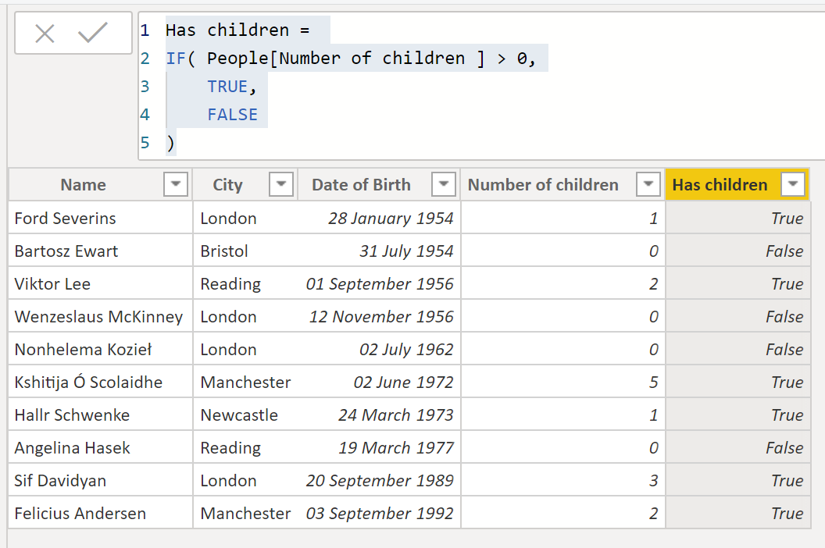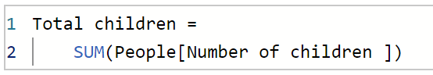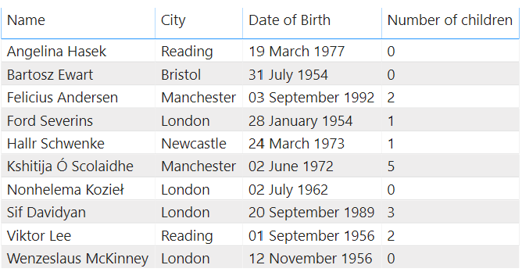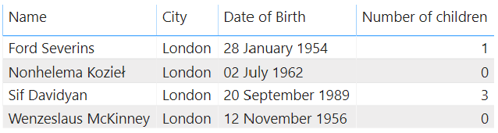So, in my previous 2 blogs I went over the theory of filter contexts and row contexts, now it's time for some examples!

## Calculated columns

I have already touched on how calculated columns work: They iterate over all of the rows of the table, using the row context to evaluate the formula for the current row.

We saw in our previous example that we could define a new column which indicates whether or not a person has children by going to the table view and clicking "new column".Here we are using an `IF` statement. The first argument is the condition to be evaluated, the second argument is the value if that condition is `TRUE`, and the third is the value if it is `FALSE`.

This expression is re-evaluated for each row every time the data is refreshed, and the values are stored in the model. Therefore each calculated column will mean that the model takes up more disk space. This is fine in our small example, but this can be important to consider as datasets get larger.

## Measures

The output of a calculated column is, well, a column. If you instead want to aggregate a column to produce a value, you need to use a measure.

We have also seen an example of this already, where we calculated the total number of children. If we click "new measure" and enter the following:We have seen that we can display the total number of children as follows:And have seen that measures operate under any filter context which has been applied:These values are evaluated every time you query the data (unlike calculated columns which are calculated on data refresh). The formula for evaluating the measure is stored on the model, but the value itself is calculated every time. This means that measures do not cause the model to take up much extra memory, but instead consume CPU.

In general, it is best practice to use measures where possible as DAX is optimized for these operations and in general reports will be memory limited rather than CPU.

## So, in summary:

We have calculated columns which are evaluated row by row for a table. The values for each row are added to the model and cause a report to consume more memory.

We have measures which are used to calculate a single valued aggregation over a column (or multiple columns). The values are not added to the model and using these cause a report to consume more CPU.

There are many different aggregation functions, and I will go into this in more detail in my next blog.

### Carmel Eve

#### Software Engineer I